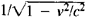Homopolar Induction

The following article is from The Great Soviet Encyclopedia (1979). It might be outdated or ideologically biased.

Homopolar Induction

(in Russian, unipoliarnaia induk-tsiia), the generation of an electromotive force (emf) in a magnetized body moving in a direction that is not parallel to the axis of magnetization. The direction of the emf is perpendicular to the plane in which the magnetic induction vector B and the magnet’s velocity vector v lie.

If the magnetized body is a conductor, homopolar induction may be explained in the framework of classical electrodynamics. Under the action of the Lorentz force, free electrons drift within the body in a direction perpendicular to the directions of v and B until an electric field that impedes the drift is generated in the body.

The theory of relativity provides a consistent explanation of homopolar induction. In the reference frame associated with the magnet, or the proper frame of reference, there is no electric field E. Suppose the magnet moves with a velocity v in the laboratory reference frame and the motion is uniform, translational, and rectilinear. Then, according to the relativistic field-strength equations, the electric field E in the laboratory frame is E = – [vB]/c, where c is the speed of light; the value of E is accurate to within the factor, which is practically equal to 1 for small values of v. The equation for the electric field is applicable both within and outside the magnetized body, regardless of whether the body is a conductor. Thus, homopolar induction is a relativistic effect in which the relative nature of the partition of an electromagnetic field into an electric field and a magnetic field is explicitly revealed.

The presence of an electric field gives rise to a constant potential difference. This phenomenon is used to generate a direct current in homopolar generators.

The term “unipolar induction,” of which the Russian term is a cognate, is deprecated in English. The term came into use because, in a homopolar generator, all the magnetic poles presented to the circuit in which the emf is induced are of the same polarity.

REFERENCE

Tamm, I. E. Osnovy teorii elektrichestva, 8th ed. Moscow, 1966.